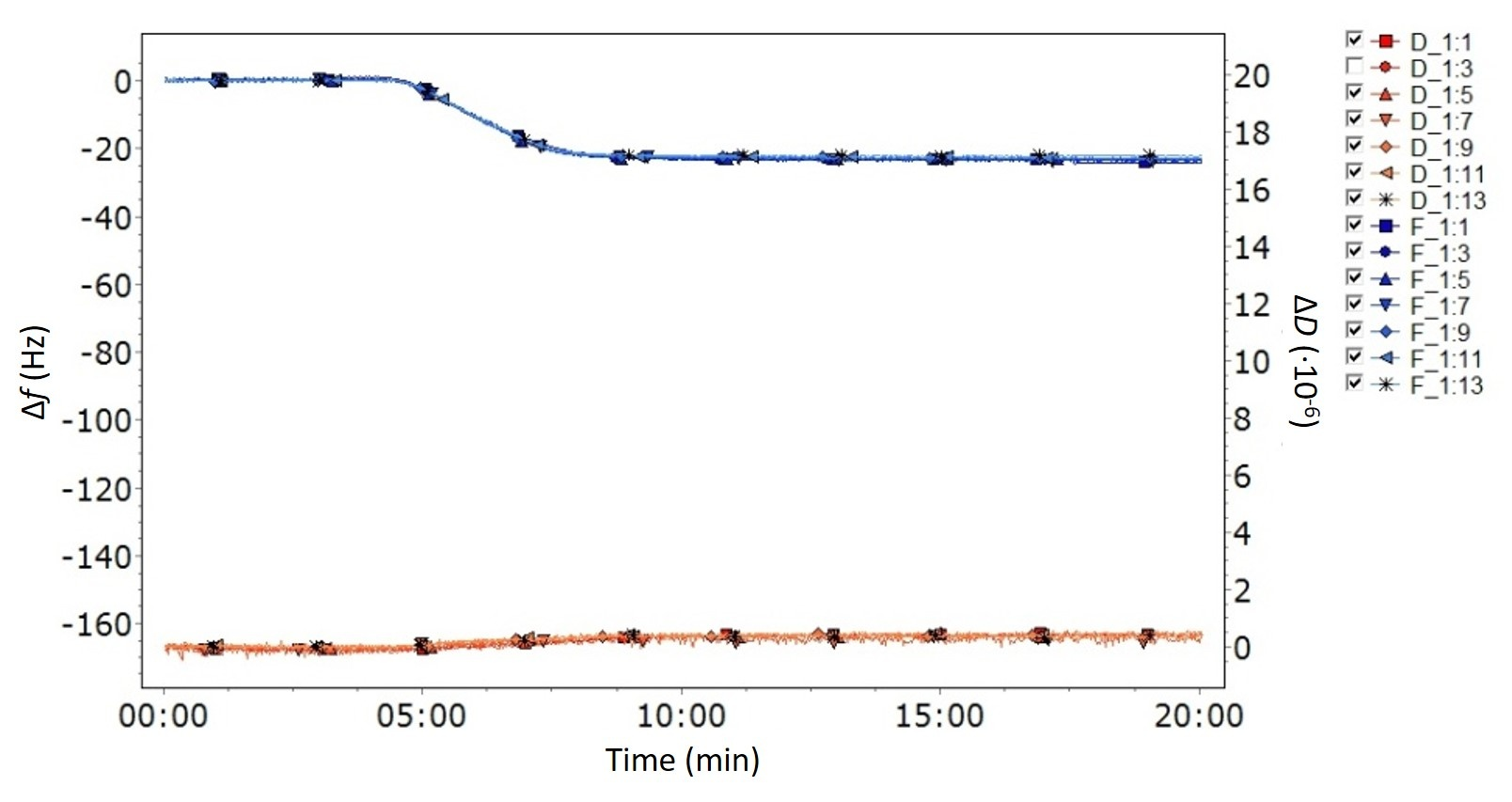Malin Edvardsson Apr 25, ’23 < 5 min

# The Sauerbrey equation or Viscoelastic modeling? – how to assess which method to use

## Surface Science Blog

Imagine a scenario where you have just finished a set of successful QCM experiments, and now it is time to analyze the data. You are mainly interested in the changes of mass and thickness so this is what you plan quantify. Now, you must decide which method to use – will it be the Sauerbrey equation or viscoelastic modeling? So, what are those rules of thumb that tell you when to use which approach? Don’t worry – here you have them - the guidelines on how to assess which method to use.

## Let the QCM-D raw data guide you

The Sauerbrey equation is valid for thin and rigid films, where the energy losses are small. If these conditions do not apply, the viscoelastic nature of your film needs to be considered and viscoelastic modeling should be used. So how can you tell if you stand before one situation or the other? The key is to look at the Δf and ΔD data, i. e., changes in recorded frequency and dissipation, respectively. Ideally these should be recorded for multiple harmonics of the QCM.

## When to use the Sauerbrey equation

If the change in energy loss is recorded, you can tell that the layer is rigid and that the energy losses are small by the fact that ΔD is close to zero, Fig. 1. Another key characteristic of a rigid layer is that the harmonics are overlapping. A typical scenario would be the one shown in Fig 1, where it looks like only one single harmonic has been recorded.

The Sauerbrey mass is calculated using one of the measured harmonics, and it should not matter which one is used, all harmonics should provide the same answer. If the harmonics overlap, the magnitude of the mass will not depend on which one of the harmonics that is used for the calculation.

#### Use Sauerbrey when:

• ΔD ~ 0 for all harmonics
• Δf for all harmonics overlapFigure 1. A typical situation where the Sauerbrey equation should be used is identified by a low D-value and overlapping harmonics. In this graph, the curves overlap to such an extent that it looks like only one single harmonic is captured.

## When to use viscoelastic modeling

If the layer is thick and/or soft, viscoelastic modeling should be used. A viscoelastic layer is typically identified by two key characteristics in the data, i) the energy losses, ΔD, are larger than zero, and ii) the overtones are spreading. Spreading overtones reveal that the layer properties influence the recorded signals, and each harmonic will provide unique information on these layer properties.

#### Use viscoelastic modeling when:

• ΔD > 0Figure 2. A typical situation where viscoelastic modeling should be used is identified by a large D-value and spreading harmonics, where each curve is clearly separated from the others.

## Concluding remarks

If the layer to be analyzed fulfills the modeling requirements, there are two approaches that could be used to calculate the mass depending on whether the viscoelastic nature of the film needs to be considered or not. Which analysis method to use can generally be assessed by looking at key characteristics in the raw data, f and D, ideally at multiple harmonics.

Download the guide to get a summary of the guidelines on how to assess which analysis method to use.GuideEditors note: This post was originally published in 2018 and has been updated

## Explore the blog

You have only scratched the surface.